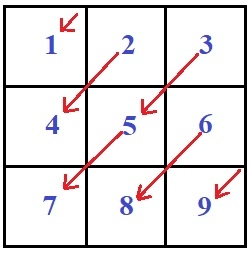Print the matrix diagonally downwards in C Program.

CServer Side ProgrammingProgramming

Given with an array of size n x n and the task is to print the matrix elements of integer type diagonally downwards.

Diagonally downwards means printing the array of any size of n x n in diagonally moving downward like in the figure given below −Firstly it will print 1 and then move to 2 print it and moves down to 4 diagonally and print it and so on.

Example

Input: Matrix  = {
{ 1, 2, 3 },
{ 4, 5, 6 },
{ 7, 8, 9 }}
Output: 1 2 4 3 5 7 6 8 9

Algorithm

int diagonally_down(int mat[n][n])
START
STEP 1: DECLARE i, j, row, col AS INT
STEP 2: LOOP FOR i = 0 AND i < n AND i++
SET row = 0
SET col = i
LOOP WHILE col >= 0
PRINT mat[row][col]
INCREMENT row BY 1 AND DECREMENT col BY 1
END WHILE
END FOR
STEP 3: LOOP FOR j = 1 AND j < n AND j++
SET row = j
SET col = n-1
LOOP WHILE row < n
PRINT mat[row][col]
INCREMENT row BY 1 AND DECREMENT col BY 1
END WHILE
END FOR
STOP

Example

#include <stdio.h>
#define n 3
int diagonally_down(int mat[n][n]){
int i, j, row, col;
//printing above elements
for (i = 0; i < n; i++){
row = 0;
col = i;
while(col >= 0) //Moving downwards from the first row{
printf("%d ", mat[row++][col--]);
}
}
//printing below elements
for (j = 1; j < n; j++){
row = j;
col = n-1;
while(row<n) //Moving from the last column{
printf("%d ", mat[row++][col--]);
}
}
}
int main(int argc, char const *argv[]){
int mat[][n] = {
{1, 2, 3},
{4, 5, 6},
{7, 8, 9}
};
diagonally_down(mat);
return 0;
}

Output

If we run above program then it will generate following output −

1 2 4 3 5 7 6 8 9